Computer Science

# General functions and images

This is another joint lecture/lab! We’ll do some things in class together, and you’ll do some coding in groups.

Our goal is to draw a border around an image, like this:We’ll use this example to learn about how to write general-purpose functions.

⚠️ Always download the image directly from Unsplash or Pexels. Don’t right click and save it.

## Top border

Let’s start by drawing a border on the top. We might call this function:

``def draw_top_border():``
• What parameters should this function take?

### Generality

What if you want the same function to be able to draw a 20-pixel border that has the color of the sky?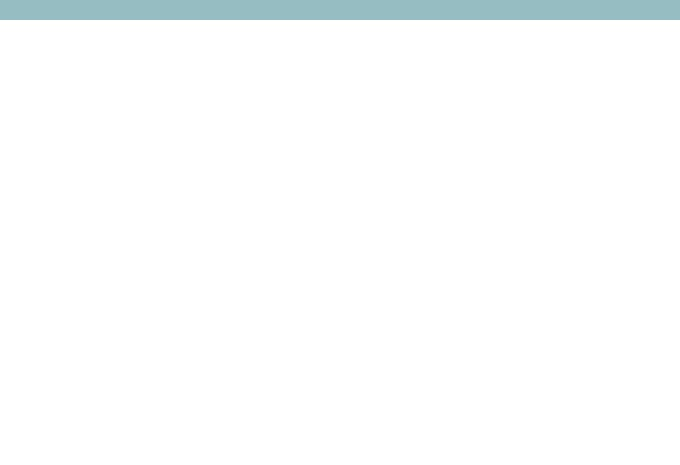But also a 50-pixel border that has the color of the sand?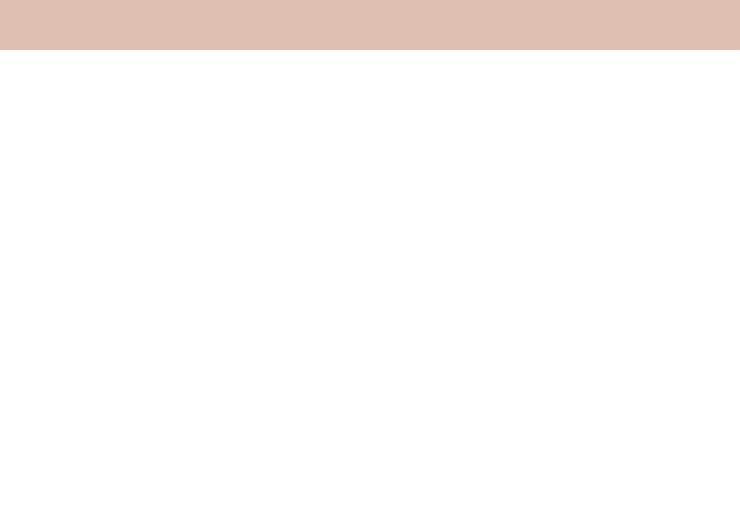Think about what you want to be able to change inside the function. For example, we might want to be able to change the height of the border and its color:

``def draw_top_border(image, height, red, green, blue):``

Notice how we also want to be able to change the image that we apply this to.

### Writing the function

Once you have the parameters figured out, you can then write the function:

``````def draw_top_border(image, height, red, green, blue):
for y in range(height):
for x in range(image.width):
pixel = image.get_pixel(x, y)
pixel.red = red
pixel.green = green
pixel.blue = blue``````

Notice the following:

• we use `range(height)` because we want the border to be drawn in rows `0...height`
• we use `range(image.width)` because we want the border to be drawn in all columns from `0...image.width`
• we can copy in the red, green, and blue values of the pixel from the function parameters

This function, like many we have written, loops through the rows (using `y`) first, and then through the columns (using `x`). First, `y` will have the value 0, and then `x` will get the values `0..image.width`. So for an image that is 100 pixels wide, we will first work on pixel `(0,0)`, then `(1,0)`, `(2,0)``(99,0)`, and then `(0, 1)`, `(1,1)`…and so on.

You could write this function with the columns first:

``````def draw_top_border(image, height, red, green, blue):
for x in range(image.width):
for y in range(height):
pixel = image.get_pixel(x, y)
pixel.red = red
pixel.green = green
pixel.blue = blue``````

This would loop through the columns first (using `x`) and then the rows (using `y`). First, `x` will have the value 0, and then `y` will get the values `0..image.height`. So for an image that is 50 pixels high, we will first work on pixel `(0,0)`, then `(0,1)`, `(0,2)``(0,49)`, and then `(1,0)`, `(1,1)`… and so on.

### Using the function

You can use this function to see how it works:

``````def image_with_top_border():
height = 20
red = 150
green = 189
blue = 194
new_image = Image.blank(800, 600)
draw_top_border(new_image, height, red, green, blue)
new_image.show()

image_with_top_border()``````

Try changing the height and color!

## Generalizing the border function

How can we take what we’ve learned and generalize this? What if you wanted a single function that could draw a bar anywhere?

``def draw_bar():``

### Defining the function

• What parameters should this function take?
• It should be able to draw a stripe, either vertical or horizontal, anywhere on an image

For example, what about these cases?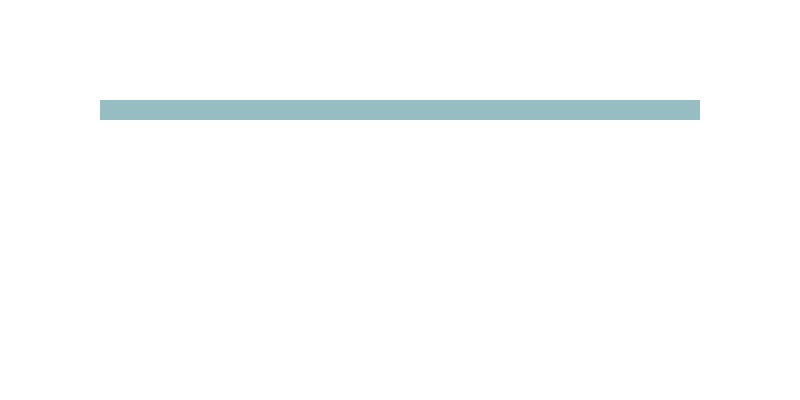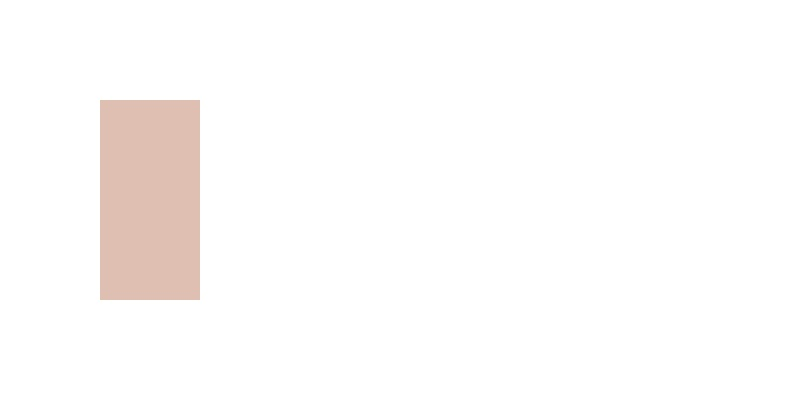The first step is to draw out what you need: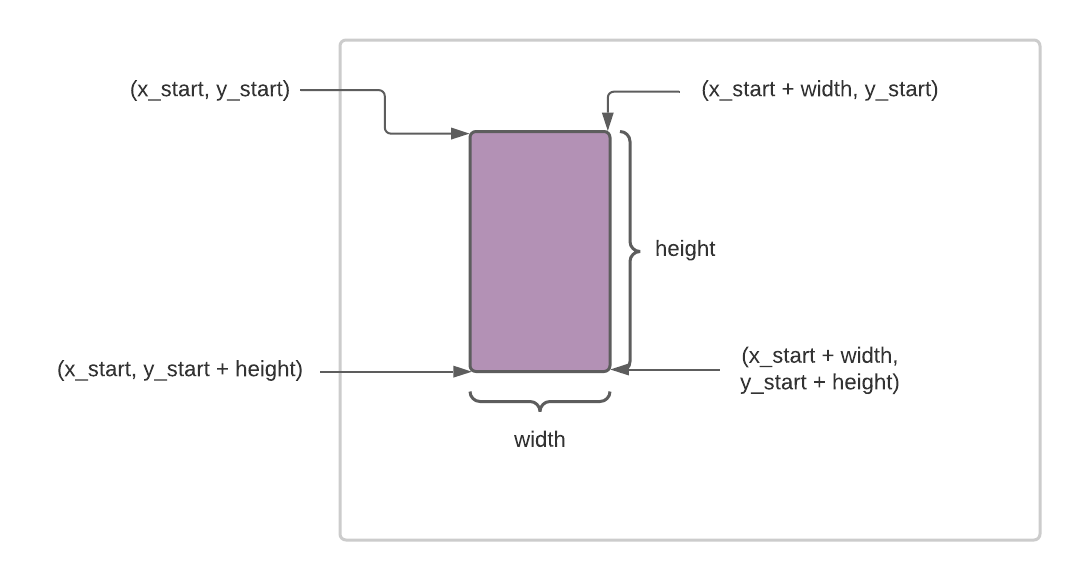The key here is to include parameters that control the starting `(X, Y)` of the bar as well as its width and height:

``def draw_bar(image, x_start, y_start, width, height, red, green, blue):``

### Writing the function

Once you have the parameters figured out, you can then write the function:

``````def draw_bar(image, x_start, y_start, width, height, red, green, blue):
for y in range(height):
for x in range(width):
pixel = image.get_pixel(x_start + x, y_start + y)
pixel.red = red
pixel.green = green
pixel.blue = blue``````

Notice the following:

• We use `x_start` and `y_start` to define the starting position of the bar.
• We use `width` and `height` to define how big the bar is.
• Inside the function, we can use `(x_start + x, y_start + y)` draw a pixel for the bar, with `(x, y)` being controlled by the nested for loops.
• This function is not that much longer than the one that just draws the border on top! This is the power of generality, your code can often be concise.

### Using range()

So far, we have only used `range()` to count up from 0 to some maximum value. So `range(100)` counts from 0 to 99. You can actually specify a starting value for `range()` as well, so `range(10,20)` counts from 10 to 19.

This means we could rewrite `draw_bar()` as:

``````def draw_bar(image, x_start, y_start, width, height, red, green, blue):
for y in range(y_start, y_start + height):
for x in range(x_start, x_start + width):
pixel = image.get_pixel(x, y)
pixel.red = red
pixel.green = green
pixel.blue = blue``````

or, reversing the `x` and `y` loops:

``````def draw_bar(image, x_start, y_start, width, height, red, green, blue):
for x in range(x_start, x_start + width):
for y in range(y_start, y_start + height):
pixel = image.get_pixel(x, y)
pixel.red = red
pixel.green = green
pixel.blue = blue``````

This may be more intuitive to you. We can see from the `range()` functions that we are looping over the `x` values from `x_start` to `x_start + width` and the `y` values from `y_start` to `y_start + height`. This is precisely the area the bar covers.

### Using the function

Using this function is similar as before:

``````def image_with_bar():
width = 100
height = 200
red = 150
green = 189
blue = 194
new_image = Image.blank(800, 400)
draw_bar(new_image, 100, 100, width, height, red, green, blue)
new_image.show()

image_with_bar()``````

Try changing the height and color!

## Drawing borders

Now, can you use our bar function to draw borders at the top, bottom, left, and right of an image? You want a function:

``def make_borders(image, thickness, red, green, blue):``

That can make borders on all sides of an image, using the given `thickness` and colors. This function should call `draw_bar().

Once you have written this function, you can call it like this:

``````def image_with_borders():
thickness = 50
red = 150
green = 189
blue = 194
new_image = Image.blank(800, 600)
make_borders(new_image, thickness, red, green, blue)
new_image.show()``````

that can produce something like this (using a 50 pixel width):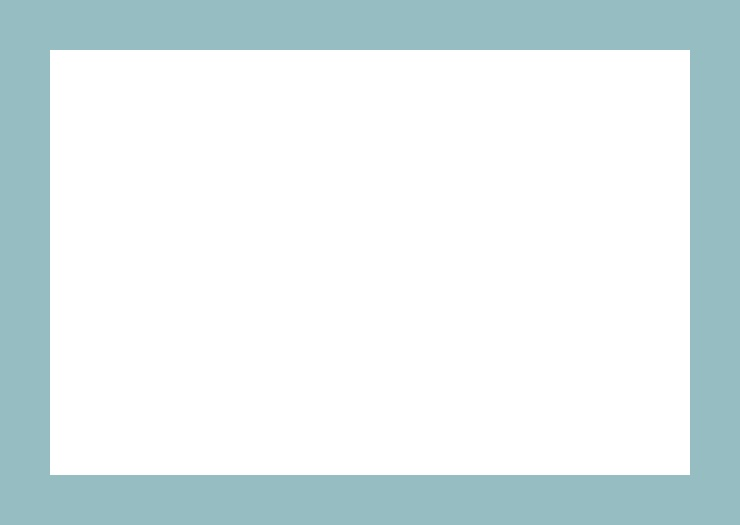## Copying the image

The last step is to copy an image into the space that has been reserved in the middle of the borders. We want a function that is general enough to work for many situations. So you need a function like this:

``def copy_image(image, image_to_copy, x_start, y_start):``

The `image` parameter is the image you are copying into (which could have borders for example) and the `image_to_copy` parameter is the image you want to copy into this one. Just like the `draw_bar()` function, this function uses `x_start` and `y_start` to indicate where the copied image should be placed.

Once you write this function you can use it like this:

``````def photo_with_borders(filename):
thickness = 20
red = 150
green = 189
blue = 194
photo = Image(filename)
new_image = Image.blank(photo.width + 2*thickness, photo.height + 2*thickness)
make_borders(new_image, thickness, red, blue, green)
copy_image(new_image, photo, thickness, thickness)
new_image.show()

photo_with_borders("sea.jpg")``````

Notice that we create a blank image with enough space to hold the borders. Then we make the borders and finally copy in the image.

You should end up with this:Try different border sizes and colors, as well as different photos.